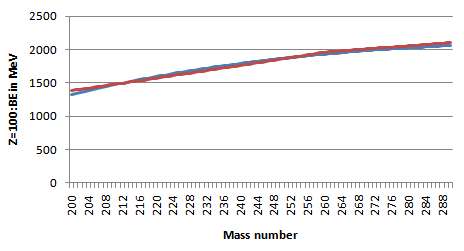## Figures index

#### U. V. S. Seshavatharam, S. Lakshminarayana

Frontiers of Astronomy, Astrophysics and Cosmology. 2015, 1(1), 43-55• Figure 1. Binding energy at mean stable mass numbers of Z=2 to 100• Figure 2. Binding energy of isotopes of Z=26• Figure 3. Binding energy of isotopes of Z=40• Figure 4. Binding energy of isotopes of Z=60• Figure 5. Binding energy of isotopes of Z=80• Figure 6. Binding energy of isotopes of Z=100